Courses

# Linear Programming (Part - 2) Electronics and Communication Engineering (ECE) Notes | EduRev

## Electronics and Communication Engineering (ECE) : Linear Programming (Part - 2) Electronics and Communication Engineering (ECE) Notes | EduRev

The document Linear Programming (Part - 2) Electronics and Communication Engineering (ECE) Notes | EduRev is a part of Electronics and Communication Engineering (ECE) category.
All you need of Electronics and Communication Engineering (ECE) at this link: Electronics and Communication Engineering (ECE)

Linear Programming - Part - 2, Operations Research

SOLVING LP

LP Solutions: Four Cases

When an LP is solved, one of the following four cases will occur:
1. The LP has a unique optimal solution.
2. The LP has alternative (multiple) optimal solutions. It has more than one (actually an infinite number of) optimal solutions
3. The LP is infeasible. It has no feasible solutions (The feasible region contains no points).
4. The LP is unbounded. In the feasible region there are points with arbitrarily large (in a max problem) objective function values.

The Graphical Solution

Any LP with only two variables can be solved graphically

Example 1. Giapetto

(Winston 3.1, p. 49)
Since the Giapetto LP has two variables, it may be solved graphically.

Answer

The feasible region is the set of all points satisfying the constraints.

max z = 3x1 + 2x2
s.t.
2x1 + x2 ≤ 100   (Finishing constraint)
x1 + x2 ≤ 80    (Carpentry constraint)
x1 ≤ 40            (Demand constraint)
x1, x2 ≥ 0        (Sign restrictions)

The set of points satisfying the LP is bounded by the five sided polygon DGFEH. Any point on or in the interior of this polygon (the shade area) is in the feasible region. Having identified the feasible region for the LP, a search can begin for the optimal solution which will be the point in the feasible region with the largest z-value (maximization problem).

To find the optimal solution, a line on which the points have the same z-value is graphed. In a max problem, such a line is called an isoprofit line while in a min problem, this is called the isocost line. (The figure shows the isoprofit lines for z = 60, z = 100, and z = 180).

In the unique optimal solution case, isoprofit line last hits a point (vertex - corner) before leaving the feasible region.

The optimal solution of this LP is point G where (x1, x2) = (20, 60) giving z = 180.

A constraint is binding (active, tight) if the left-hand and right-hand side of the constraint are equal when the optimal values of the decision variables are substituted into the constraint.

A constraint is nonbinding (inactive) if the left-hand side and the right-hand side of the constraint are unequal when the optimal values of the decision variables are substituted into the constraint.

In Giapetto LP, the finishing and carpentry constraints are binding. On the other hand the demand constraint for wooden soldiers is nonbinding since at the optimal solution x< 40 (x1 = 20).

Example 2. Advertisement

(Winston 3.2, p. 61)

Since the Advertisement LP has two variables, it may be solved graphically.

Answer

The feasible region is the set of all points satisfying the constraints.
min z = 50x+ 100x2
s.t.
7x1 + 2x≥ 28      (high income women)
2x+ 12x2 ≥ 24     (high income men)
x1, x2 ≥ 0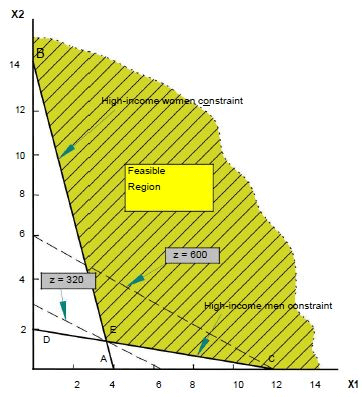Since Dorian wants to minimize total advertising costs, the optimal solution to the problem is the point in the feasible region with the smallest z value.
An isocost line with the smallest z value passes through point E and is the optimal solution at x1 = 3.6 and x2 = 1.4 giving z = 320.
Both the high-income women and high-income men constraints are satisfied, both constraints are binding.

Example 3. Two Mines

min 180x + 160y
st 6x + y ≥ 12
3x + y ≥ 8
4x + 6y ≥ 24
x ≤ 5
y ≤ 5
x, y ≥ 0

Answer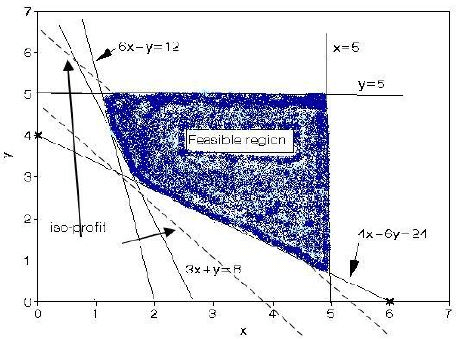Optimal sol’n is 765.71. 1.71 days mine X and 2.86 days mine Y are operated.

Example 4. Modified Giapetto

max z = 4x1 + 2x2
s.t.
2x+ x2 ≤ 100      (Finishing constraint)
x1 + x2 ≤ 80         (Carpentry constraint)
x1 ≤ 40                 (Demand constraint)
x1, x2 ≥ 0             (Sign restrictions)

Answer

Points on the line between points G (20, 60) and F (40, 20) are the alternative
optimal solutions (see figure below).
Thus, for 0 ≤ c ≤ 1,
c [20 60] + (1 - c) [40 20] = [40 - 20c, 20 + 40c] will be optimal
For all optimal solutions, the optimal objective function value is 200.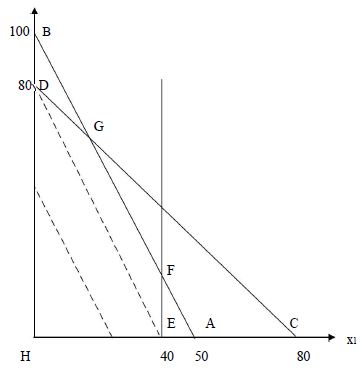Example 5. Modified Giapetto (v. 2)

Add constraint x2 ≥ 90 (Constraint on demand for trains).

Answer

No feasible region: Infeasible LP

Example 6. Modified Giapetto (v. 3)

Only use constraint x2 ≥ 90

Answer

Isoprofit line never lose contact with the feasible region: Unbounded LP

The Simplex Algorithm

Note that in the examples considered at the graphical solution, the unique optimal solution to the LP occurred at a vertex (corner) of the feasible region. In fact it is true that for any LP the optimal solution occurs at a vertex of the feasible region. This fact is the key to the simplex algorithm for solving LP's.

Essentially the simplex algorithm starts at one vertex of the feasible region and moves (at each iteration) to another (adjacent) vertex, improving (or leaving unchanged) the objective function as it does so, until it reaches the vertex corresponding to the optimal LP solution.

The simplex algorithm for solving linear programs (LP's) was developed by Dantzig in the late 1940's and since then a number of different versions of the algorithm have been developed. One of these later versions, called the revised simplex algorithm (sometimes known as the "product form of the inverse" simplex algorithm) forms the basis of most modern computer packages for solving LP's.

Steps

1. Convert the LP to standard form
2. Obtain a basic feasible solution (bfs) from the standard form
3. Determine whether the current bfs is optimal. If it is optimal, stop.
4. If the current bfs is not optimal, determine which nonbasic variable should become a basic variable and which basic variable should become a nonbasic variable to find a new bfs with a better objective function value
5. Go back to Step 3.

Related concepts:

• Standard form: all constraints are equations and all variables are nonnegative
• bfs: any basic solution where all variables are nonnegative
• Nonbasic variable: a chosen set of variables where variables equal to 0
• Basic variable: the remaining variables that satisfy the system of equations at the standard form

Example 1. Dakota Furniture

(Winston 4.3, p. 134)
Dakota Furniture makes desks, tables, and chairs. Each product needs the limited resources of lumber, carpentry and finishing; as described in the table. At most 5 tables can be sold per week. Maximize weekly revenue.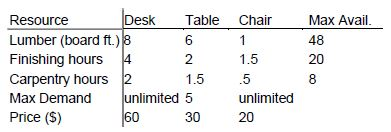LP Model:

Let x1, x2, x3 be the number of desks, tables and chairs produced.
Let the weekly profit be \$z. Then, we must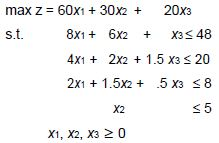Solution with Simplex Algorithm

First introduce slack variables and convert the LP to the standard form and write a canonical form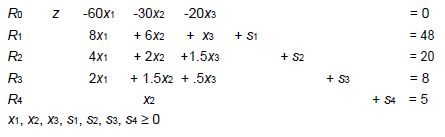Obtain a starting bfs.

As (x1, x2, x3) = 0 is feasible for the original problem, the below given point where three of the variables equal 0 (the non-basic variables) and the four other variables (the basic variables) are determined by the four equalities is an obvious bfs:
x= x2 = x3 = 0, s1 = 48, s= 20, s3 = 8, s4 = 5.
.
Determine whether the current bfs is optimal.

Determine whether there is any way that z can be increased by increasing some nonbasic variable.
If each nonbasic variable has a nonnegative coefficient in the objective function row (row 0), current bfs is optimal.
However, here all nonbasic variables have negative coefficients: It is not optimal.

Find a new bfs

• z increases most rapidly when x1 is made non-zero; i.e. x1 is the entering variable.
• Examining R1, x1 can be increased only to 6. More than 6 makes s1 < 0. Similarly R2, R3, and R4, give limits of 5, 4, and no limit for x1 (ratio test). The smallest ratio is the largest value of the entering variable that will keep all the current basic variables nonnegative. Thus by R3, x1 can only increase to x1 = 4 when s3 becomes 0. We say s3 is the leaving variable and R3 is the pivot equation.
• Now we must rewrite the system so the values of the basic variables can be read off.

The new pivot equation (R3/2) is
R3’ : x1+.75x2+.25x3+ .5s3= 4

Then use R3’ to eliminate x1 in all the other rows.

R0’=R0+60R3’, R1’=R1-8R3’, R2’=R2-4R3’, R4’=R4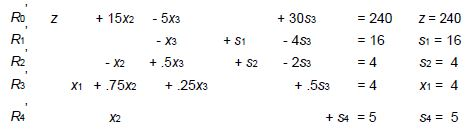The new bfs is x2 = x3 = s3 = 0, x1 = 4, s1 = 16, s2 = 4, s4 = 5 making z = 240.

Check optimality of current bfs. Repeat steps until an optimal solution is reached

• We increase z fastest by making x3 non-zero (i.e. x3 enters).
• x3 can be increased to at most x3 = 8, when s2 = 0 ( i.e. s2 leaves.)

Rearranging the pivot equation gives
R2’’ - 2x2 + x3 + 2s2 - 4s3 = 8 (R2’ × 2).
Row operations with R2’’ eliminate x3 to give the new system
R0’’= R0’ + 5R2’’, R1’’ = R1’ + R2’’, R3’’ = R3’ - .5R2’’, R4’’ = R4
The bfs is now x2 = s2 = s3 = 0, x1 = 2, x3 = 8, s1 = 24, s4 = 5 making z = 280.
Each nonbasic variable has a nonnegative coefficient in row 0 (5x2, 10s2, 10s3).

THE CURRENT SOLUTION IS OPTIMAL

Report: Dakota furniture’s optimum weekly profit would be 280\$ if they produce 2 desks and 8 chairs.

This was once written as a tableau.
(Use tableau format for each operation in all HW and exams!!! )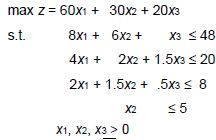Initial tableau: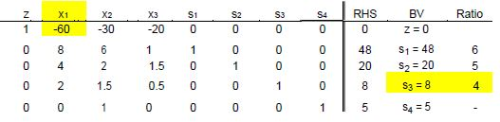First tableau: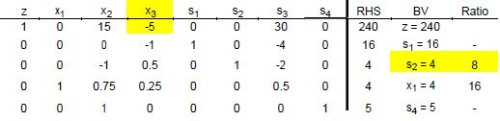Second and optimal tableau: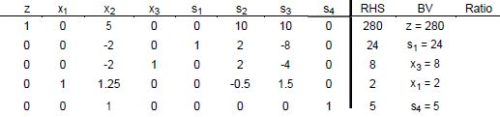Example 2. Modified Dakota Furniture

Dakota example is modified: \$35/table

new z = 60 x1 + 35 x+ 20 x3

Second and optimal tableau for the modified problem: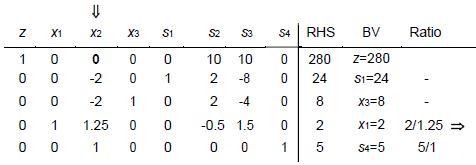Another optimal tableau for the modified problem: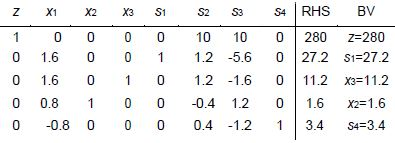Therefore the optimal solution is as follows: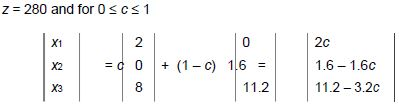Example 3. Unbounded LPs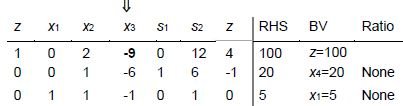Since ratio test fails, the LP under consideration is an unbounded LP.

Offer running on EduRev: Apply code STAYHOME200 to get INR 200 off on our premium plan EduRev Infinity!

,

,

,

,

,

,

,

,

,

,

,

,

,

,

,

,

,

,

,

,

,

;